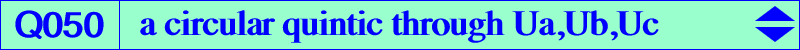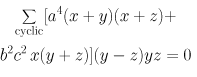X(2), X(3), X(4), X(13), X(14), X(67), X(1113), X(1114) midpoints of ABC Ga, Gb, Gc vertices of the antimedial triangle A', B', C' projections of O on the sidelines of the antimedial triangle points Ua, Ub, Uc mentioned in the Neuberg cubic page. See also table 16 and table 18. points at infinity of the Thomson cubic foci of the inconic with perspector X(76), center X(141) anti-points, see Table 77Q050 is a circular quintic with singular focus G. It has three real asymptotes parallel to those of the Thomson cubic. A, B, C are double points on the curve. Q050 is the locus of point M such that G, M and the isogonal conjugate of M with respect to the circumcevian triangle of M (or the inverse in (O) of the isogonal conjugate of M) are collinear. The isogonal transform of Q050 is Q136. *** Generalization Let P be a point. The locus of point M such that P, M and the isogonal conjugate of M with respect to the circumcevian triangle of M are collinear is in general a circular circum-quintic Q(P) passing through H, P, the vertices of the cevian triangle of P, the infinite points of pK(X6, P), the foci of the inconic with perspector tgP and center cgP, the intersections of the circumcircle and the line OP when P ≠ O (when P = O, Q(P) splits into the circumcircle and the McCay cubic). A, B, C are three nodes on Q(P). For example, Q050 = Q(X2) and Q094 = Q(X6). Special cases : Q(X4) has also a node at the orthocenter H and its real asymptotes concur at O. Q(X54) has perpendicular nodal tangents at A, B, C. When P lies on the Euler line, Q(P) contains O, X(1113), X(1114). Q(P) is bicircular when P lies on the line at infinity. *** A pencil of related quintics Q003 and the union of the line at infinity, the circumcircle, the Kiepert hyperbola generate a pencil of circular trinodal circum-quintics which contains Q050, Q135 and several other curves.